×

Search anything:

# Super Resolution GAN: SRGAN

#### Machine Learning (ML) Deep Learning## Introduction

Super Resolution imaging is referred to as using different techniques to convert a lower resolution image to higher resolution image, it is mostly performed on upsampled images. Super Resolution GAN (SRGAN) is generative adversarial network that can generate high resolution images from low resolution images using perceptual loss function that is made of the adversarial loss as well as the content loss. As we know the GAN is made of the generator and the discriminator , hence the discriminator is made to diffrentiate between the real image and the super-resolved images and the generator tries to generate super resolved images, the adversarial loss mentioned above helps in naturalising the super resolved images. The content loss on the other hand helps enhance the perceptual similarity rather than improving on the pixel space. The above perceptual similarity is again calculated by an index.

Usually the supervised super resolution algorithms focus on improving the resolution of images by reducing the Mean Squared error (MSE) and increasing the Peak Signal Noise Ratio (PSNR) , which results in improvement in pixel wise differences but perceptual differences still exist and this not necessarily represents finer texture details. In the below figure you can observe that the increase in PSNR ratio doesn't represent the high resolution image.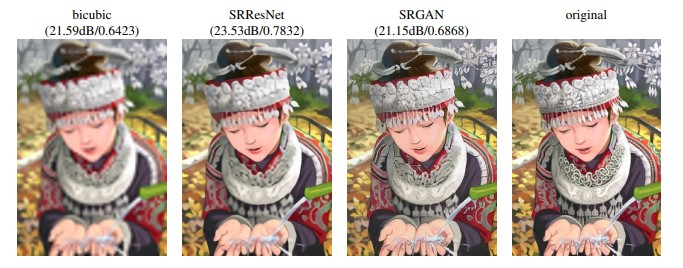In this research paper, the author focuses on mainly Single Image Super Resolution (SISR) and introduces method that avoids the smoothening effect post application , unlike that of filtering methods.

## Design/ Architecture of SRGAN

According to the research of the authors on different literatures it was seen that deep neural networks produce high accuracy even though it was hard to train deep networks.To efficiently train these networks batch normalization was supposed to be performed in order to prevent internal covariate shifts.

In this paper , the authors propose a new architecture that uses a 16 block deep ResNet that performs high upscaling (4x) that also replaces the MSE based optimization and prevents the smoothening effect. The architecture not to forget is a GAN based architecture , meaning that there is the generator to generate photo realistic images and the discriminator to differentiate between the generated and the original images.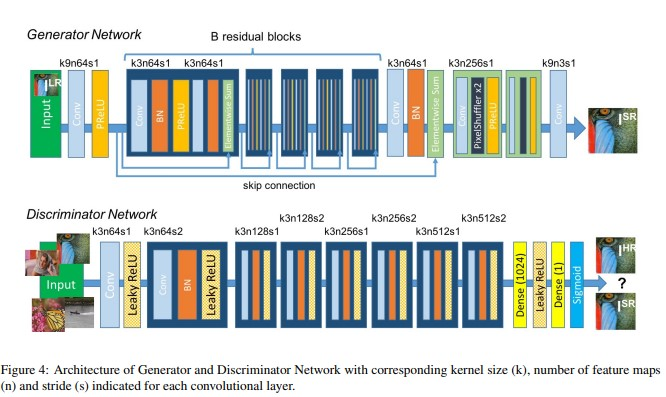The generator network is a feed forword CNN that has the main goal of converting a Low Resolution image to a High Resolution image by a SR specific loss function. The generator is made of B Residual blocks and the generator performs the following operations in each residual block:

• Input: The input given to the generator of GAN is the Low Resolution Image.
• Convolution block : Convolutions are done in this block wherein there are 64 , 3x3 filters that help in extracting features from the input.
• Batch Normalization : Post feature extraction the batch of dataset is normalized to lesser the computation and prevent internal covariate shifts.
• PReLU: The generator also uses the PReLu or the Parametric ReLu as the activation function which further helps to deal with the Negative values and prevents the dead neuron problem, the parametric ReLU multiplies the result in negative by a parameter alpha=0.01 .
• PixelShuffler: The PixelShuffler is another component in the generator network that helps in reshaping a tensor recieved by a shuffling factor, such that the image could be upsampled and no resolution is lost unlike in the strided convolutions where such resolutions could be lost.
• Output: The ouput is the SR Image.

The discriminator network performs the following operations in order:

• Convolutional block : There are 8 convolutional blocks with the filter size 3 X 3.
• Batch Normalization: Discriminator performs batch normalization to reduce computational complexity.
• LeakyReLU: Makes use of the LeakyReLU activation function. LeakyReLu has a small slope for the negative values and hence it avoids the zero slope error, which helps the negative values to be taken into consideration.
• Dense : To convert and connect tensor feature maps back to 1D array the dense block is used.
• Sigmoid Function: As the last classifier sigmoid is used to classify the output into either HR or SR by converting the tensors into a logical number that shows the probability of the image being HR or SR.
• Output: The output is the class of the input i.e either HR or SR.

Loss Functions:

When the MSE loss function is used the main aim is to reduce the mean squared error and in the process there are multiple potential solutions of fine textured images that are created that further gets averaged to get a smoothened image.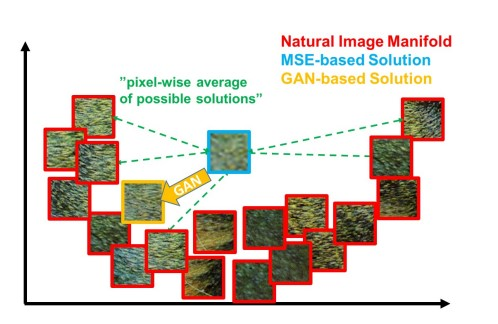The authors make use of two loss functions to develop a perceptual loss that is capable of diffrentiaing based on perceptually relevant characteristics.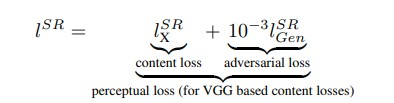Content loss:
Different from calculating MSE Loss that produces smotthened effect and ignores the high frequency features like texture ,the authors develop a loss function that not just relies on pixel wise loss but defines the VGG loss as the euclidean distance between the feature representations.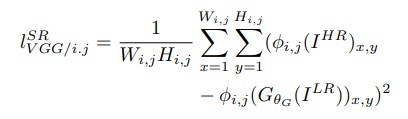The adversarial loss forces the generator to generate highly realistic images that can help fooling the discriminator.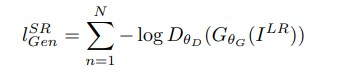Evaluation Metrics:

• PSNR: The Peak Signal-to-Noise Ratio is the ratio between the maximum power the signal can produced to the noise that distors the actual representation of the image.PSNR is usually denoted in decibels(dB).
• SSIM: The Structural Similarity Index Measure (SSIM) is used to measure the similarity between two images and this index ranges between -1 to 1. It is comparitive measure between two pixels about how similar they are.
• MOS: The Mean Opinion Score is a rating given by raters where it ranges from 1 to 5 , where 1 represents low resolved images and 5 represents highly resolved images.

Experiment:
The experiment was performed on the Set5 , Set 14 and BSD100 , the teting set BSD300. For a fair comparison all images were performed with constant upscaling factor that is 4X and the comparisons were made based on PSNR and SSIM. The training was done using the NVIDIA Tesla M40 GPU on a 350 thousand image from ImageNet database. As for the optimization algorithm the Adam was used.

Mean Opinion Score (MOS) Testing:
According to this Test there were 26 raters that rated 12 versions of each image. In this case the SRGAN performs greatly and shows a good score of nearly 3.5 on BSD100.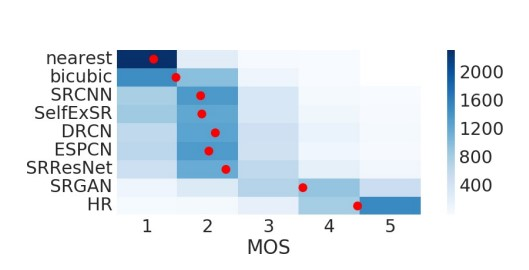The PSNR , SSIM and the MOS rating altogether can be seen in the below table where as For the PSNR and the SSIM the SRResNet performs exceptionally well but when it comes to the MOS Rating as discussed above the SRGAN plays a better role.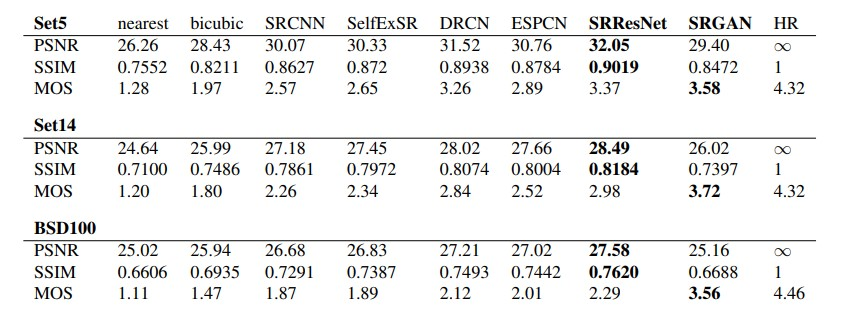The paper also further goes to show that the standard measures i.e the PSNR and the SSIM fail to assess the image quality and hence contradictory to HR images the LR images get higher PSNR and SSIM score.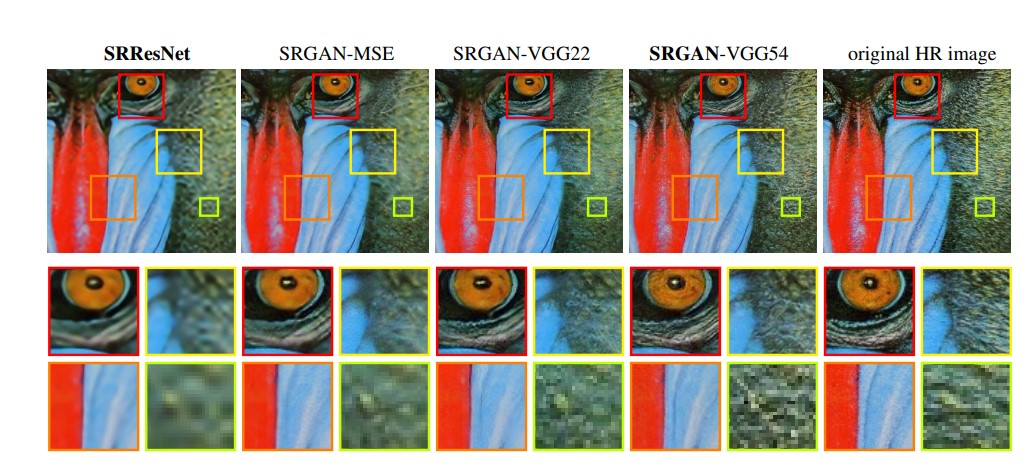FAQ's:

1. What are pixels?
Soln: A pixel is the smallest unit of a digital image and is also known as picture element , a pixel is usually of one shade of a color, the amount of colors could be defined by the number of bits given to the digital device . For example , if 2 bits are given to the system , there could be 4 combinations of colors between black and white namely, black,white,light grey and dark grey.

2. What is the smoothening effect?
Soln: Smoothing is a technique used to reduce noises in an image, during smoothing the neighbouring pixels are either averaged or maxed , this generally leads to the LR of edges in an image.

3. How does the generator generate images?
Soln : The generator takes noise from latent space and further based on test and trial feedback , the generator keeps improvising on images. After a certain times of trial and error , the generator starts producing accurate images of a certain class which are quite difficult to diffrentiate from real images.

4. How does the discriminator perform classification?
Soln: The discriminator gets a probability score after convolutions and hence the discriminator chooses the decision based on the probability.

#### Anubhav Tewari

Anubhav Tewari has been a Machine Learning Developer, Intern at OpenGenus.

Super Resolution GAN: SRGAN